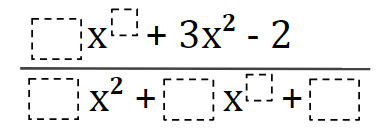# L’Hospital’s Rule Exploration

Directions: Using the digits 1 to 9, at most one time each, create 3 different expressions such that their graphs contains any 2 of the 3 following criteria:

1) Horizontal Asymptote @ y = some positive rational number
2) Slant Asymptote with a slope such that: 1 < m ≤ 2
3) Two Vertical Asymptotes### Hint

Top choices vary as they are limited by bottom choices {bot: 8,1,7}

Is it possible to get a positive and negative asymptote with our restrictions?

Vert Asymptote: First term exponent < 2; Set bottom = Zero Slant Asymptote: First term exponent > 2; Slope set by coefficients of first terms simplified

Horiz. Asymptote: First term exponent = 2: Location by coefficients of first terms simplified

All the above hold true because LARGEST term exponent on bottom is “”2″”